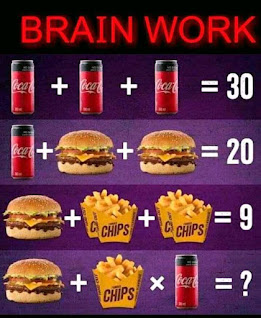0

# Answer to Coca Cola, hamburger and chips banana math puzzleAnswer to Coca Cola, hamburger and chips banana math puzzle

Welcome to www.cammeroonhowto.com where I solve tricky math puzzles, quizzes, riddles and more with detailed explanations. Today I will solve the " Coca Cola, hamburger and chips banana Puzzle for you."

This is one of those tricky math  puzzles that keeps many people scratching their heads on the internet, especially on Twitter, Pinterest and Facebook.  When the Puzzle was published on Facebook, many people solved it and had different opinions and answers. Majority had 17, 70, 25 and 30, all wrong.

Solving Math Puzzles can be interesting and funny. Be sure to share this one with loved ones.

## Hamburger +a packet of Chip × Coca-Cola = ?

The correct answer would be 15 not 17, 70 or  30, the trick in the fruit math Puzzle is found in the last equation because in the last equation, there's only one packet of chips and you need to apply BODMAS carefully too.

Many game lovers like solving math puzzles suduku, quizzes like this one to refresh their brain.

Here's the puzzle extraction:

If

Coca-Cola + Coca Cola + Coca-Cola  = 30,

Coca cola + Hamburger + Hamburger = 20

Hamburger + 2packet chips + 2packet chips= 9

Then Hamburger + a packet of chips × Coca-Cola =?

Due to the Corona various pandemic, many people isolate themselves because of lockdowns and to avoid contracting or spreading the deadly disease.

Isolation can lead to boredom. One way to refresh the brain, relax and get busy is  by solving riddles, puzzles and Quizzes.

I will form four equations, solve equation 1, 2 and three mathematically and then substitute answers in the final equation and simplify.

Equation one

3cocacola =30

Implies Coca-Cola   = 30÷3,

Coca-Cola= 10.

Equation two

10 + 2hamburger = 20

Implies 2hambuger = 20 minus 10

Hamburger = 5

Equation three

5+ 2 packet chips + 2 packet chips = 9,

4 packet chips= 9 minus 5

Packet chips = 1

Therefore, the value of Coca Cola, hamburger and chips banana are as Thus:

Coca-Cola = 10

hamburger= 5

Packet of chips = 1

Remember you can still get all the values by simply counting but it's important to use math rules in case of larger numbers.

Final equation

I will substitute the values of the variables above in the final equation below and simplify.

## Hamburger + a packet of chips × Coca-Cola =?

5 + 1 × 10 = ? ( Apply BODMAS)

5 + 10= ?

15 = ?

So the correct answer to the tricky  math Puzzle "Hamburger + a packet of chips × Coca-Cola =?

=?"  Is

= 15.

If you don't apply BODMAS, you will get 30 which is a mathematically wrong answer.

Do you have another way to arrive at this Answer? Share with us.

Don't forget to test your friends, family members and loved ones by sharing the puzzle.